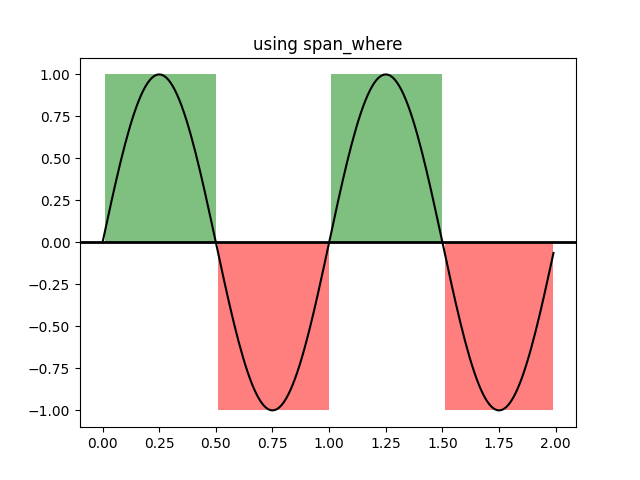# Using span_where¶

Illustrate some helper functions for shading regions where a logical mask is True.

import numpy as np
import matplotlib.pyplot as plt
import matplotlib.collections as collections

t = np.arange(0.0, 2, 0.01)
s1 = np.sin(2*np.pi*t)
s2 = 1.2*np.sin(4*np.pi*t)

fig, ax = plt.subplots()
ax.set_title('using span_where')
ax.plot(t, s1, color='black')
ax.axhline(0, color='black', lw=2)

collection = collections.BrokenBarHCollection.span_where(
t, ymin=0, ymax=1, where=s1 > 0, facecolor='green', alpha=0.5)

collection = collections.BrokenBarHCollection.span_where(
t, ymin=-1, ymax=0, where=s1 < 0, facecolor='red', alpha=0.5)